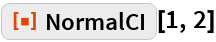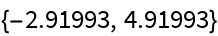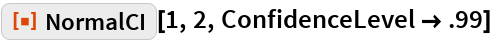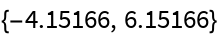#Function Repository Resource:

# NormalCI

Estimate the confidence interval based on a normal distribution

Contributed by: Wolfram Research
 ResourceFunction["NormalCI"][μ,σ] gives a confidence interval based on a normal distribution.

## Details and Options

ResourceFunction["NormalCI"][μ,σ] gives a confidence interval {min,max} centered at μ based on a normal distribution with location parameter μ and scale parameter σ.
ResourceFunction["NormalCI"] is the confidence interval for a population mean based on a sample mean μ and standard error σ if the true population variance is known.
The following option can be given:
 ConfidenceLevel 0.95 probability associated with a confidence interval

## Examples

### Basic Examples

A 95% confidence interval based on the normal distribution:

 In:=Out=### Options

#### ConfidenceLevel

A 99% confidence interval:

 In:=Out=## Requirements

Wolfram Language 11.3 (March 2018) or above